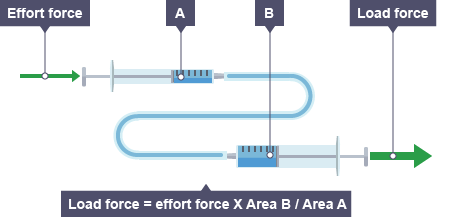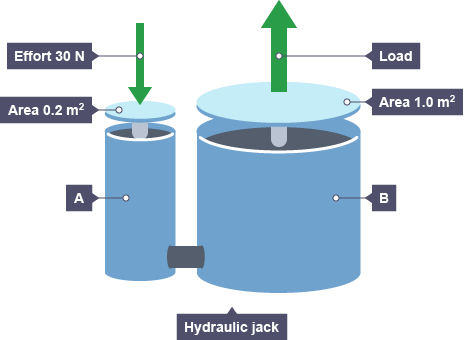### Quick Quote

• Please select the sector that most closely matches your own from the list below.
• Our independence in a global marketplace gives you choice on pricing and delivery. If you have a tough deadline to meet, we'll do all that we can to help. How quickly do you need your equipment to be dispatched?

• Drop files here or
Max. file size: 5 MB, Max. files: 3.

# Hydraulics for Beginners

### Hydraulic engineering is based on the laws of science governing pressure in liquids and the fact that hydraulic systems allow smaller forces to be multiplied into bigger forces…

Particles in liquids are close together, meaning that liquids are virtually incompressible, i.e. cannot be compacted or squashed together. When moving around the particles collide with one another and the walls of the container in which the liquid is housed.

Pressure and forces can be spread, through liquids with the pressure transmitted equally in all directions. So, a force applied at one point on a liquid will spread equally to other points in the liquid. The French mathematician, Blaise Pascal discovered and established this fact, which is now known as “Pascal’s Law”.

So, if you had a bucket filled with water, but the bucket had holes in it, then the water leaking from each hole would give out equal pressure.

Pressure is calculated using the equation:

Pressure (P) = Force (F) ÷ Cross-Sectional Area (A)

Where:

P = Pressure in pascals (Pa),

F = Force in newtons (N)

A = Cross-sectional area in metres squared, m2

For example: A force of 100N is applied over an area of 5m2.  What is the pressure?

P = F ÷ A

P = 100 ÷ 5

P = 20 Pa

### Hydraulic Engineering

Pressure in a liquid is transmitted equally in all directions. This means that when a force is applied to one point of the liquid, it will spread to other points within the liquid. Hydraulic engineering is based on, and exploits, this principle and the fact that when exerting a small force over a small cross-sectional area, pressure can be transmitted to create a large force over a large cross-sectional area.

Imagine that two syringes of different sizes were connected by tubing and filled with water.An “effort force” pushing on syringe “A” increases the pressure on the water in syringe tube “A”. As water is virtually incompressible, the pressure spreads through the water into syringe “B”. The water pushes against the plunger in syringe “B” with equal pressure, exerting a “load force” onto it.

However, syringe B has a plunger with a bigger cross-sectional area than syringe “A”. This means that the “load force” exerted is larger than the original “effort force” that was exerted on syringe “A”. This is known as a “force multiplier”. Note, however, that the bigger syringe moves a shorter distance than the smaller syringe.

Hydraulic systems therefore allow smaller forces to be multiplied into bigger forces. Car braking systems provide a perfect example of the application of this in everyday life.

We can look at the diagram here of a hydraulic jack and calculate the force on piston B.Step 1: First we need to calculate the pressure of the liquid inside piston A, so remembering the earlier equation for calculating pressure:

Pressure (P) = Force (F) ÷ Cross-Sectional Area (A)

Where: P = Pressure in pascals (Pa), F = Force in newtons (N) and A = Cross-sectional area in metres squared, m2

P = 30 ÷ 0.2

P = 150 Pa

We then need to move on to find the force in Piston B – but remember that the pressure within this closed system is transmitted equally in all directions, therefore the pressure in piston B is also 150 Pa.

Pressure (P) = Force (F) ÷ Cross-Sectional Area (A)

Therefore:

Force (F) = Pressure (P) x Cross-Sectional Area (A)

But the cross-sectional area in piston B = 1.0 m2 and so,

F = 150 x 1.0

In this example, the hydraulic jack can lift load forces five times greater than the effort force put in.

### Applications of Hydraulics

Hydraulic systems allow smaller forces to be multiplied into bigger forces. Car braking systems provide a perfect example of the application of this in everyday life.

It takes a large force to slow down or stop a car that is travelling at speed. Hydraulics are used in the braking system of a car; they cause a relatively small force from the driver’s foot to be multiplied to produce a greater force, which acts equally on all four brake pads.The force from the driver’s foot (the effort force) puts pressure on the brake fluid in a small piston. The pressure is transmitted throughout the brake fluid in all directions.

Next to each brake disc, there is a much larger piston with a greater cross-sectional area. The transmitted pressure acts on this larger area to produce a larger load force on the the brake pads. The pads then rub against the brake discs and cause the car to slow down.

Hydraulic systems are everywhere around us and also found in: fairground rides, lifting equipment (e.g. passenger and goods lifts, diggers, dentist chairs) winches on fishing boats, wind turbines, in factories, security gates and barriers, commercial vehicle tail lifts, horse boxes, piling rigs and aircraft including the droop nose on ConcordeThe list goes on and on…